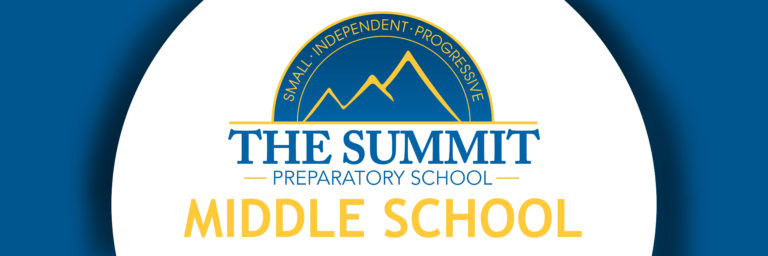### Middle School Mathematics

As we are nearing the midpoint of the school year, math students often feel the pressure of increasing complexity and difficulty in math concepts. With

### Middle School Mathematics

Math Fundamentals  Sixth grade students worked with multiplying and dividing fractions. The algorithms for performing these operations aren’t difficult, so most of our focus was

### Middle School Mathematics

Math Fundamentals  Fractions and decimals are our key focus for the next few weeks. Students will practice the procedures for operations with fractions and decimals.

### Middle School Mathematics

Math Fundamentals  Percentages are everywhere! Sixth grade students are learning how to work with percentages in a variety of contexts, including sales, comparing amounts, and

### Middle School Mathematics

What’s with all the problems? In Upper School math classes, students are quite often presented with challenging real-world and/or hands-on problems to work out. Typically

### Middle School Mathematics

Math Fundamentals  Students have been exploring with lots of ratios. They have been (digitally) mixing paint, making multiple batches of recipes, and determining the best

### Middle School Mathematics

Math Fundamentals  This week students finished up their study of the coordinate plane and adding and subtracting integers. Students had many opportunities to visualize the

### Middle School Mathematics

As we begin the month of September, mathematics classes are in full swing. Students are approaching or have already completed their first math assessments. The

### Middle School Mathematics

What’s with all the whiteboards? In The Summit math classes, students are frequently seen clustered around small whiteboards on the walls. This work has been

### Middle School Mathematics

Math Fundamentals – This week sixth graders took a self-paced approach to learning about mean and mean absolute deviation. We are studying measures of center